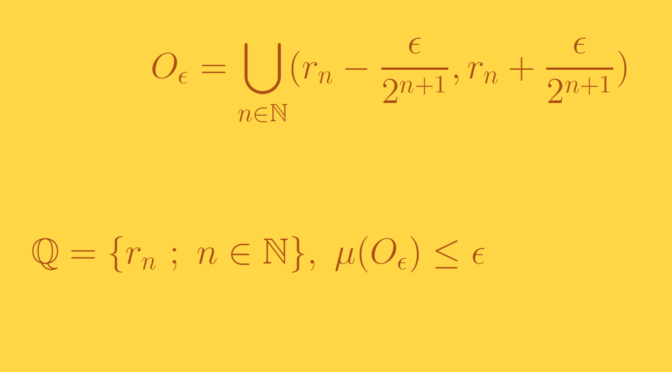# Small open sets containing the rationals

The set $$\mathbb Q$$ of the rational number is countable infinite and dense in $$\mathbb R$$. You can have a look here on a way to build a bijective map between $$\mathbb N$$ and $$\mathbb Q$$.

Now given $$\epsilon > 0$$, can one find an open set $$O_\epsilon$$ of measure less than $$\epsilon$$ with $$\mathbb Q \subseteq O_\epsilon$$?

The answer is positive. Let’s denote $O_\epsilon = \bigcup_{n \in \mathbb N} (r_n – \frac{\epsilon}{2^{n+1}},r_n + \frac{\epsilon}{2^{n+1}})$ where $$(r_n)_{n \in \mathbb N}$$ is an enumeration of the rationals. Obviously $$\mathbb Q \subseteq O_\epsilon$$. Using countable subadditivity of Lebesgue measure $$\mu$$, we get:
\begin{align*}
\mu(O_\epsilon) &\le \sum_{n \in \mathbb N} \mu((r_n – \frac{\epsilon}{2^{n+1}},r_n + \frac{\epsilon}{2^{n+1}}))\\
&= \sum_{n \in \mathbb N} \frac{2 \epsilon}{2^{n+1}} = \sum_{n \in \mathbb N} \frac{\epsilon}{2^n} = \epsilon
\end{align*}

• The open segments $$(r_n – \frac{\epsilon}{2^{n+1}},r_n + \frac{\epsilon}{2^{n+1}})$$ are overlapping. Hence $$\mu(O_\epsilon)$$ is strictly less than $$\epsilon$$.